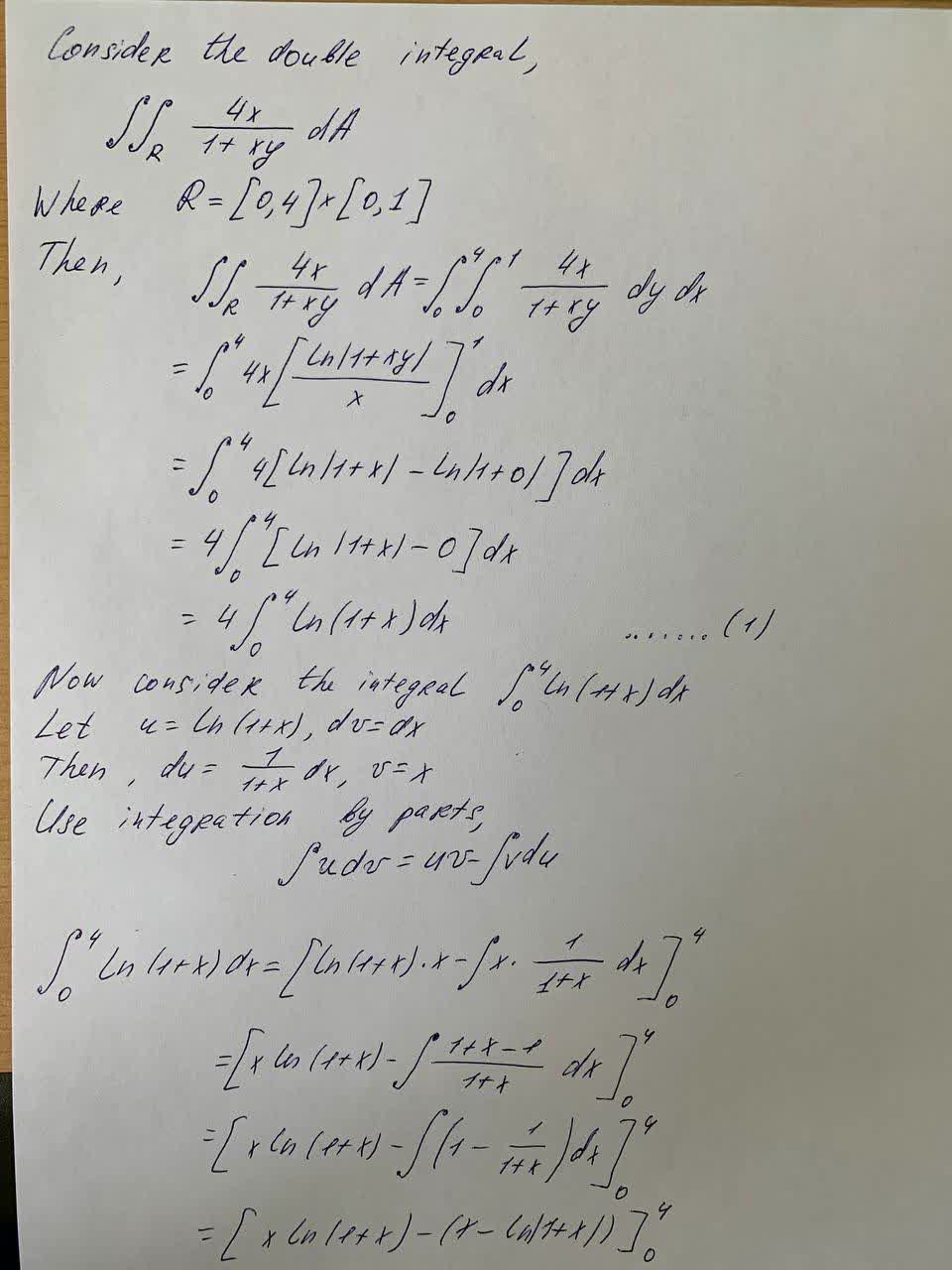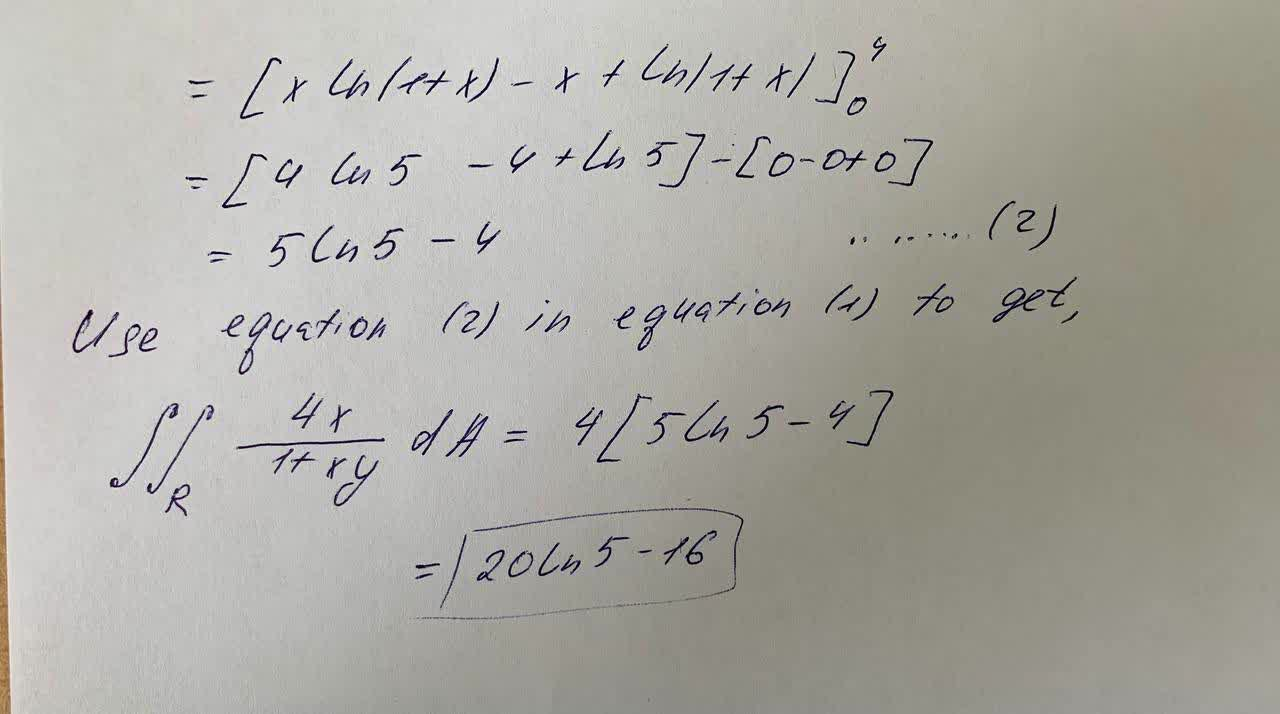# Calculate the double integral. \int \int_R \frac{4x}{1+xy}dA R=\left[0,4UkusakazaL 2021-09-21 Answered
Calculate the double integral.
$$\displaystyle\int\int_{{R}}{\frac{{{4}{x}}}{{{1}+{x}{y}}}}{d}{A}$$
$$\displaystyle{R}={\left[{0},{4}\right]}\times{\left[{0},{1}\right]}$$

• Questions are typically answered in as fast as 30 minutes

### Plainmath recommends

• Get a detailed answer even on the hardest topics.
• Ask an expert for a step-by-step guidance to learn to do it yourself.svartmaleJ
Calculation: